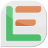# Support Forum

### Approximating a Circle with Polygons

Jim
LayoutEditorFull
Monday 25th March 2019
Hi Jürgen, In LayoutEditor, instead of approximating a circle with an inscribed polygon, is it possible to have a circumscribed polygon around the circle (where the flats of the polygon, not the vertices, touch the circle)? Jim with PhotomaskPORTAL
Jürgen
LayoutEditorFull
Monday 25th March 2019
Hi Jim, to archive that just the radius needs to be adjusted. The new radius had to be calculated with: ``` r' = r / cos (180/n) ``` with ***n*** number of points used for the circle and ***r*** the inner radius It should be no problem to add an option for this calculation in the circle properties dialog.
Jim
LayoutEditorFull
Thursday 28th March 2019
Attachments:
(only for registered users)Example_of_Rotated_Circles.lec
Hi Jürgen, That sounds great. As long as you are working on the circle properties dialog box, another option that would be useful is to rotate the vertices by 180/n so that four of the edges are axis-aligned on the top and sides of the polygonal approximation. So the vertices would no longer be on the primary axes but the midpoint of the edges would be. Jim with PhotomaskPORTAL
Jim
LayoutEditorFull
Wednesday 3rd April 2019
Hi Jürgen, I appreciate the additions you made to the Circle Dialog box in the last upgrade of LayoutEditor (20190401). Thank you. Rotating a single dodecagon in place by 180 / n (where n is the number of vertices), the macro commands are ``` layout->drawing->point(0,0); layout->drawing->rotate(15.000000); ``` So given a cell (or selection) with several polygons (circles) that each has a different -- but always even -- number of vertices, what would the macro look like to cycle through the individual shapes and rotate the individual polygons in place around their centers by 180 / n degrees? Can you steer me in the right direction? Thanks, Jim with PhotomaskPORTAL
Jürgen
LayoutEditorFull
Thursday 4th April 2019
Hi Jim, this macro will rotate any circle in the design in a way that the most right edge is upright: ```C++ #!/usr/bin/layout #name=circle rotate #help=rotate any circle in the design to an upright edge int main() { // get first cell cellList *cells=layout->drawing->firstCell; point pCenter; int radius; // loop over all cells while (cells!=NULL){ if (cells->thisCell!=NULL){ // get first Element of this cell elementList *l=cells->thisCell->firstElement; cells->thisCell->deselectAll(); // loop over all elements while (l!=NULL) { if (l->thisElement!=NULL) { // find circles (regular polygons with >8 vertices) if ( l->thisElement->isCircle(&pCenter,&radius) ){ pointArray pa=l->thisElement->getPoints(); int max=-1e10; int pos=-1; //find maximum x coordinate for (int i=0;i<pa.size()-1;++i){ if (pa.point(i).x()>max)) { pos=i; max=pa.point(i).x(); } } // calculate angle of maximal x-edge double angle= l->thisElement->angle(pa.point(pos),pa.point(pos+1)); if (angle!=90){ // rotate if not upright l->thisElement->selectAll(); cells->thisCell->rotateSelect(90-angle,pCenter); l->thisElement->deselectAll(); } } } l=l->nextElement; } } cells=cells->nextCell; } } ```
Jim
LayoutEditorFull
Thursday 4th April 2019
Very nice, Jürgen. Works even better than I requested for regular polygons that have nine vertices or more. I added some code to align either the top edge or the right edge to a primary axis. Jim with PhotomaskPORTAL #!/usr/bin/layout #name=Circle Align with Axis #help=rotate any circle in the design to an upright edge. Circle must have at least nine vertices. int main() { cellList *cells=layout->drawing->firstCell; // get first cell point pCenter; int radius; string preferred_edge; bool align_top; double target_angle; preferred_edge=layout->getText("Input","Align TOP or RIGHT edge to axis? (T/R): ","Top"); if ((preferred_edge.left(1) == "T") || (preferred_edge.left(1) == "t")) align_top = true; else align_top = false; if (align_top) { target_angle = 180; } else { target_angle = 90; } while (cells!=NULL) // loop over all cells { if (cells->thisCell!=NULL) { elementList *l=cells->thisCell->firstElement; // get first Element of this cell cells->thisCell->deselectAll(); while (l!=NULL) // loop over all elements { if (l->thisElement!=NULL) { if ( l->thisElement->isCircle(&pCenter,&radius) ) // find circles (regular polygons with >8 vertices) { pointArray pa=l->thisElement->getPoints(); int max=-1e10; int pos=-1; for (int i=0;i<pa.size()-1;++i) //find maximum coordinate { if (align_top) { if (pa.point(i).y()>max)) { pos=i; max=pa.point(i).y(); } } else { if (pa.point(i).x()>max)) { pos=i; max=pa.point(i).x(); } } } double angle= l->thisElement->angle(pa.point(pos),pa.point(pos+1)); // calculate angle of maximum point if (angle != target_angle) // rotate if not aligned to preferred edge { l->thisElement->selectAll(); cells->thisCell->rotateSelect(target_angle - angle, pCenter); l->thisElement->deselectAll(); } } } l=l->nextElement; } } cells=cells->nextCell; } }